Home > Service

Filter by date

From:

To:

# Optical Equations - Construction of Optical Equations

Date: 2009-10-15hits: 3462
Most lenses are spherical lenses: their two surfaces are parts of the surfaces of spheres, with the lens axis ideally perpendicular to both surfaces. Each surface can be convex (bulging outwards from the lens), concave (depressed into the lens), or planar (flat). The line joining the centres of the spheres making up the lens surfaces is called the axis of the lens. Typically the lens axis passes through the physical centre of the lens, because of the way they are manufactured. Lenses may be cut or ground after manufacturing to give them a different shape or size. The lens axis may then not pass through the physical centre of the lens.

Toric or sphero-cylindrical lenses have surfaces with two different radii of curvature in two orthogonal planes. They have a different focal power in different meridians. This is a form of deliberate astigmatism. More complex are aspheric lenses. These are lenses where one or both surfaces have a shape that is neither spherical nor cylindrical. Such lenses can produce images with much less aberration than standard simple lenses.

Types of simple lenses
Lenses are classified by the curvature of the two optical surfaces. A lens is biconvex (or double convex, or just convex) if both surfaces are convex. If both surfaces have the same radius of curvature, the lens is equiconvex. A lens with two concave surfaces is biconcave (or just concave). If one of the surfaces is flat, the lens is plano-convex or plano-concave depending on the curvature of the other surface. A lens with one convex and one concave side is convex-concave or meniscus. It is this type of lens that is most commonly used in corrective lenses. If the lens is biconvex or plano-convex, a collimated or parallel beam of light travelling parallel to the lens axis and passing through the lens will be converged (or focused) to a spot on the axis, at a certain distance behind the lens (known as the focal length). In this case, the lens is called a positive or converging lens.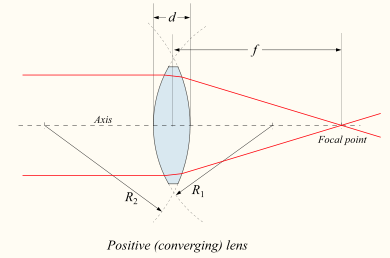If the lens is biconcave or plano-concave, a collimated beam of light passing through the lens is diverged (spread); the lens is thus called a negative or diverging lens. The beam after passing through the lens appears to be emanating from a particular point on the axis in front of the lens; the distance from this point to the lens is also known as the focal length, although it is negative with respect to the focal length of a converging lens.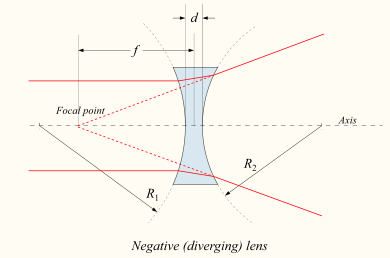Convex-concave (meniscus) lenses can be either positive or negative, depending on the relative curvatures of the two surfaces. A negative meniscus lens has a steeper concave surface and will be thinner at the centre than at the periphery. Conversely, a positive meniscus lens has a steeper convex surface and will be thicker at the centre than at the periphery. An ideal thin lens with two surfaces of equal curvature would have zero optical power, meaning that it would neither converge nor diverge light. All real lenses have a nonzero thickness, however, which affects the optical power. To obtain exactly zero optical power, a meniscus lens must have slightly unequal curvatures to account for the effect of the lens' thickness.
Lensmaker's equation
The focal length of a lens in air can be calculated from the lensmaker's equation: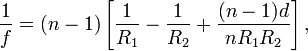where f is the focal length of the lens, n is the refractive index of the lens material, R1 is the radius of curvature of the lens surface closest to the light source, R2 is the radius of curvature of the lens surface farthest from the light source, and d is the thickness of the lens (the distance along the lens axis between the two surface vertices).
Sign convention of lens radii R1 and R2
Main article: Radius of curvature (optics) The signs of the lens' radii of curvature indicate whether the corresponding surfaces are convex or concave. The sign convention used to represent this varies, but in this article if R1 is positive the first surface is convex, and if R1 is negative the surface is concave. The signs are reversed for the back surface of the lens: if R2 is positive the surface is concave, and if R2 is negative the surface is convex. If either radius is infinite, the corresponding surface is flat. With this convention the signs are determined by the shapes of the lens surfaces, and are independent of the direction in which light travels through the lens.
Thin lens equation
If d is small compared to R1 and R2, then the thin lens approximation can be made. For a lens in air, f is then given by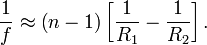The focal length f is positive for converging lenses, and negative for diverging lenses. The value 1/f is known as the optical power of the lens, measured in dioptres, which are units equal to inverse meters (m−1). Lenses have the same focal length when light travels from the back to the front as when light goes from the front to the back, although other properties of the lens, such as the aberrations are not necessarily the same in both directions
.
Imaging properties
As mentioned above, a positive or converging lens in air will focus a collimated beam travelling along the lens axis to a spot (known as the focal point) at a distance f from the lens. Conversely, a point source of light placed at the focal point will be converted into a collimated beam by the lens. These two cases are examples of image formation in lenses. In the former case, an object at an infinite distance (as represented by a collimated beam of waves) is focused to an image at the focal point of the lens. In the latter, an object at the focal length distance from the lens is imaged at infinity. The plane perpendicular to the lens axis situated at a distance f from the lens is called the focal plane. (Note: In the figure below the image is actually larger than the object; this is a function of f and S1, described below)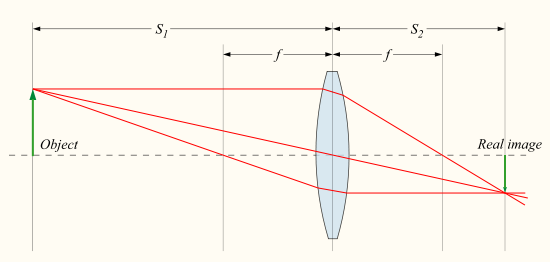If the distances from the object to the lens and from the lens to the image are S1 and S2 respectively, for a lens of negligible thickness, in air, the distances are related by the thin lens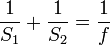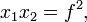What this means is that, if an object is placed at a distance S1 along the axis in front of a positive lens of focal length f, a screen placed at a distance S2 behind the lens will have a sharp image of the object projected onto it, as long as S1 > f (if the lens-to-screen distance S2 is varied slightly, the image will become less sharp). This is the principle behind photography and the human eye. The image in this case is known as a real image.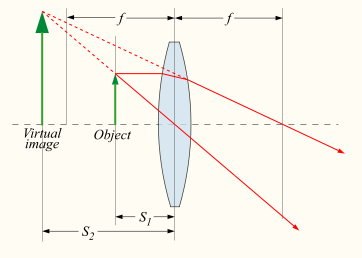Note that if S1 < f, S2 becomes negative, the image is apparently positioned on the same side of the lens as the object. Although this kind of image, known as a virtual image, cannot be projected on a screen, an observer looking through the lens will see the image in its apparent calculated position. A magnifying glass creates this kind of image. The magnification of the lens is given by:where M is the magnification factor; if |M|>1, the image is larger than the object. Notice the sign convention here shows that, if M is negative, as it is for real images, the image is upside-down with respect to the object. For virtual images, M is positive and the image is upright. In the special case that S1 = ∞, then S2 = f and M = −f / ∞ = 0. This corresponds to a collimated beam being focused to a single spot at the focal point. The size of the image in this case is not actually zero, since diffraction effects place a lower limit on the size of the image (see Rayleigh criterion).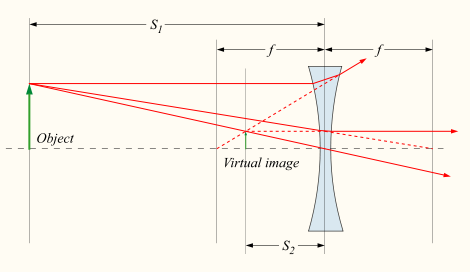The formulas above may also be used for negative (diverging) lens by using a negative focal length (f), but for these lenses only virtual images can be formed. For the case of lenses that are not thin, or for more complicated multi-lens optical systems, the same formulas can be used, but S1 and S2 are interpreted differently. If the system is in air or vacuum, S1 and S2 are measured from the front and rear principal planes of the system, respectively. Imaging in media with an index of refraction greater than 1 is more complicated, and is beyond the scope of this article.
Aberrations
Main article: Aberration in optical systems Lenses do not form perfect images, and there is always some degree of distortion or aberration introduced by the lens which causes the image to be an imperfect replica of the object. Careful design of the lens system for a particular application ensures that the aberration is minimized. There are several different types of aberration which can affect image quality.
Spherical aberration
Spherical aberration occurs because spherical surfaces are not the ideal shape with which to make a lens, but they are by far the simplest shape to which glass can be ground and polished and so are often used. Spherical aberration causes beams parallel to, but distant from, the lens axis to be focused in a slightly different place than beams close to the axis. This manifests itself as a blurring of the image. Lenses in which closer-to-ideal, non-spherical surfaces are used are called aspheric lenses. These were formerly complex to make and often extremely expensive, but advances in technology have greatly reduced the manufacturing cost for such lenses. Spherical aberration can be minimised by careful choice of the curvature of the surfaces for a particular application: for instance, a plano-convex lens which is used to focus a collimated beam produces a sharper focal spot when used with the convex side towards the beam source.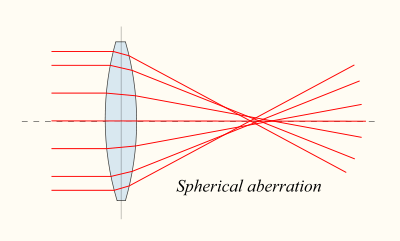Coma
Another type of aberration is coma, which derives its name from the comet-like appearance of the aberrated image. Coma occurs when an object off the optical axis of the lens is imaged, where rays pass through the lens at an angle to the axis θ. Rays which pass through the centre of the lens of focal length f are focused at a point with distance f tan θ from the axis. Rays passing through the outer margins of the lens are focused at different points, either further from the axis (positive coma) or closer to the axis (negative coma). In general, a bundle of parallel rays passing through the lens at a fixed distance from the centre of the lens are focused to a ring-shaped image in the focal plane, known as a comatic circle. The sum of all these circles results in a V-shaped or comet-like flare. As with spherical aberration, coma can be minimised (and in some cases eliminated) by choosing the curvature of the two lens surfaces to match the application. Lenses in which both spherical aberration and coma are minimised are called bestform lenses.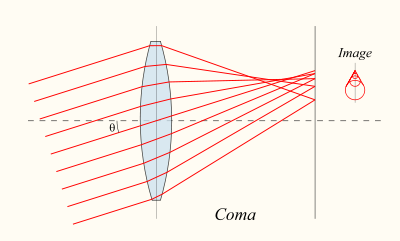Chromatic aberration
Chromatic aberration is caused by the dispersion of the lens material—the variation of its refractive index n with the wavelength of light. Since, from the formulae above, f is dependent upon n, it follows that different wavelengths of light will be focused to different positions. Chromatic aberration of a lens is seen as fringes of colour around the image. It can be minimised by using an achromatic doublet (or achromat) in which two materials with differing dispersion are bonded together to form a single lens. This reduces the amount of chromatic aberration over a certain range of wavelengths, though it does not produce perfect correction. The use of achromats was an important step in the development of the optical microscope. An apochromat is a lens or lens system which has even better correction of chromatic aberration, combined with improved correction of spherical aberration. Apochromats are much more expensive than achromats. Different lens materials may also be used to minimize chromatic aberration, such as specialized coatings or lenses made from the crystal fluorite. This naturally occurring substance has the highest known Abbe number, indicating that the material has low dispersion.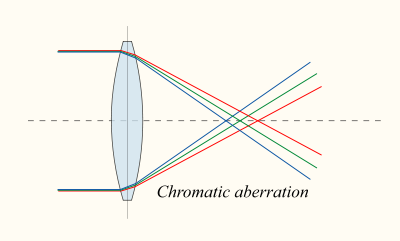Other types of aberration
Other kinds of aberration include field curvature, barrel and pincushion distortion, and astigmatism.
Aperture diffraction
Even if a lens is designed to minimize or eliminate the aberrations described above, the image quality is still limited by the diffraction of light passing through the lens' finite aperture. A diffraction-limited lens is one in which aberrations have been reduced to the point where the image quality is primarily limited by diffraction under the design conditions.
Compound lenses
See also: Photographic lens, Doublet (lens), and Achromat Simple lenses are subject to the optical aberrations discussed above. In many cases these aberrations can be compensated for to a great extent by using a combination of simple lenses with complementary aberrations. A compound lens is a collection of simple lenses of different shapes and made of materials of different refractive indices, arranged one after the other with a common axis. The simplest case is where lenses are placed in contact: if the lenses of focal lengths f1 and f2 are "thin", the combined focal length f of the lenses is given by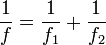Since 1/f is the power of a lens, it can be seen that the powers of thin lenses in contact are additive. If two thin lenses are separated in air by some distance d, the focal length for the combined system is given by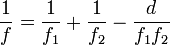The distance from the second lens to the focal point of the combined lenses is called the back focal length (BFL).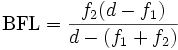As d tends to zero, the value of the BFL tends to the value of f given for thin lenses in contact. If the separation distance is equal to the sum of the focal lengths (d = f1+f2), the combined focal length and BFL are infinite. This corresponds to a pair of lenses that transform a parallel (collimated) beam into another collimated beam. This type of system is called afocal, since it produces no net convergence or divergence of the beam. Two lenses at this separation form the simplest type of optical telescope. Although the system does not alter the divergence of a collimated beam, it does alter the width of the beam. The magnification of such a telescope is given by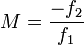which is the ratio of the input beam width to the output beam width. Note the sign convention: a telescope with two convex lenses (f1 > 0, f2 > 0) produces a negative magnification, indicating an inverted image. A convex plus a concave lens (f1 > 0 > f2) produces a positive magnification and the image is upright.